# Telling Time to the Minute: Before and After the Hour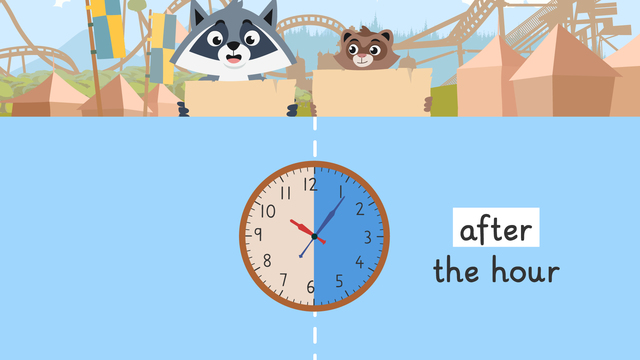Rate this video

Ø 4.5 / 2 ratings

The authorTeam Digital
Telling Time to the Minute: Before and After the Hour
CCSS.MATH.CONTENT.4.MD.A.1

## Information about the videoTelling Time to the Minute: Before and After the Hour

### Contents

• How to Tell Time to the Minute using Before the Hour and After the Hour
• ### How to Tell Time to the Minute using Before the Hour and After the Hour

Zuri and Freddie have just arrived at the Bee’s Knees Adventure Park and are trying to decide which live show to see. Zuri thinks the first show time is at 11:53 but Freddie points out that you can use the expressions before the hour and after the hour to tell time to the minute. Why is it important to tell time to the nearest minute? Since there are a few different ways to tell time, is it important to know how to tell time to the nearest minute in every way possible. How do you know which expression to use when you tell and write time to the nearest minute? In the following, we will look at exactly how this works.

#### After the Hour

Since there are 60 minutes in one hour, we can divide the clock in half at the 30 minute mark. After the hour means how many minutes have passed in the hour. We use the expression after the hour to tell time when the minute hand is on the right side of the clock.

Once you identify the minute hand on the right side of the clock, you use skip counting to count the minutes that have passed in the hour. Lastly, you determine the hour by identifying the last number the hour hand has passed.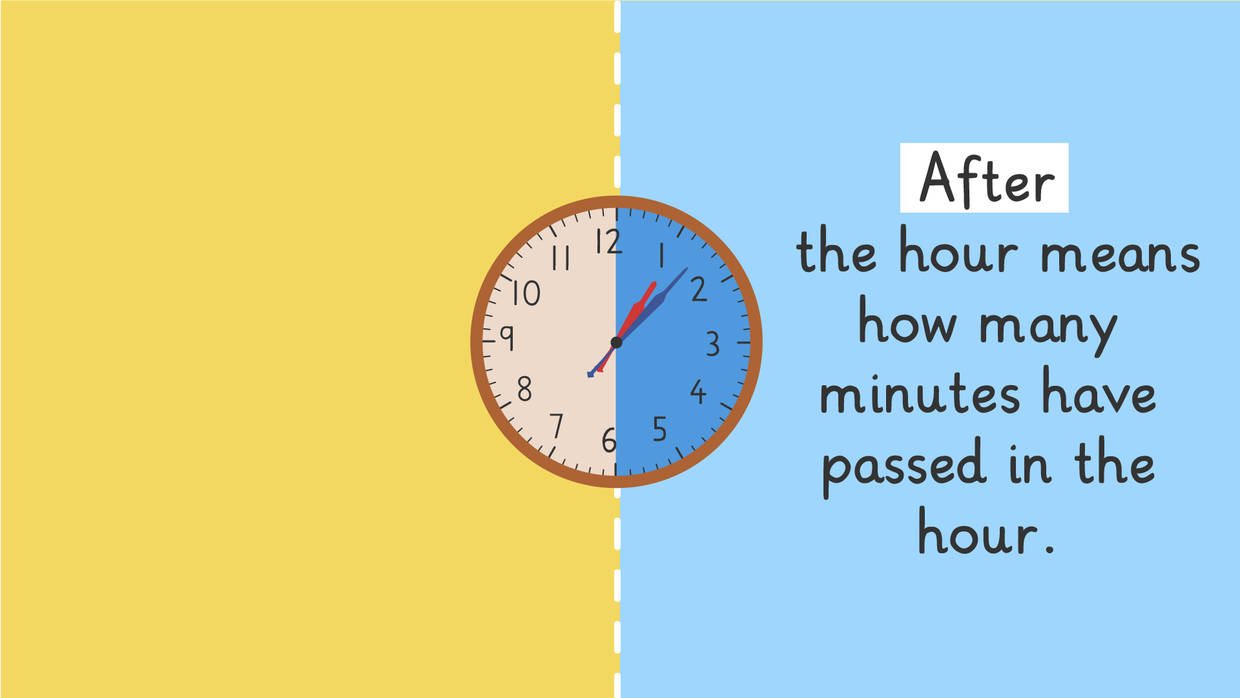#### Before the Hour

Before the hour means how many minutes are left before the next hour. We use the expression before the hour to tell time when the minute hand is on the left side of the clock.

Once you identify the minute hand on the left side of the clock, you use skip counting to count how many minutes are left before the next hour. Lastly, you determine the hour by identifying the last number the hour hand has passed.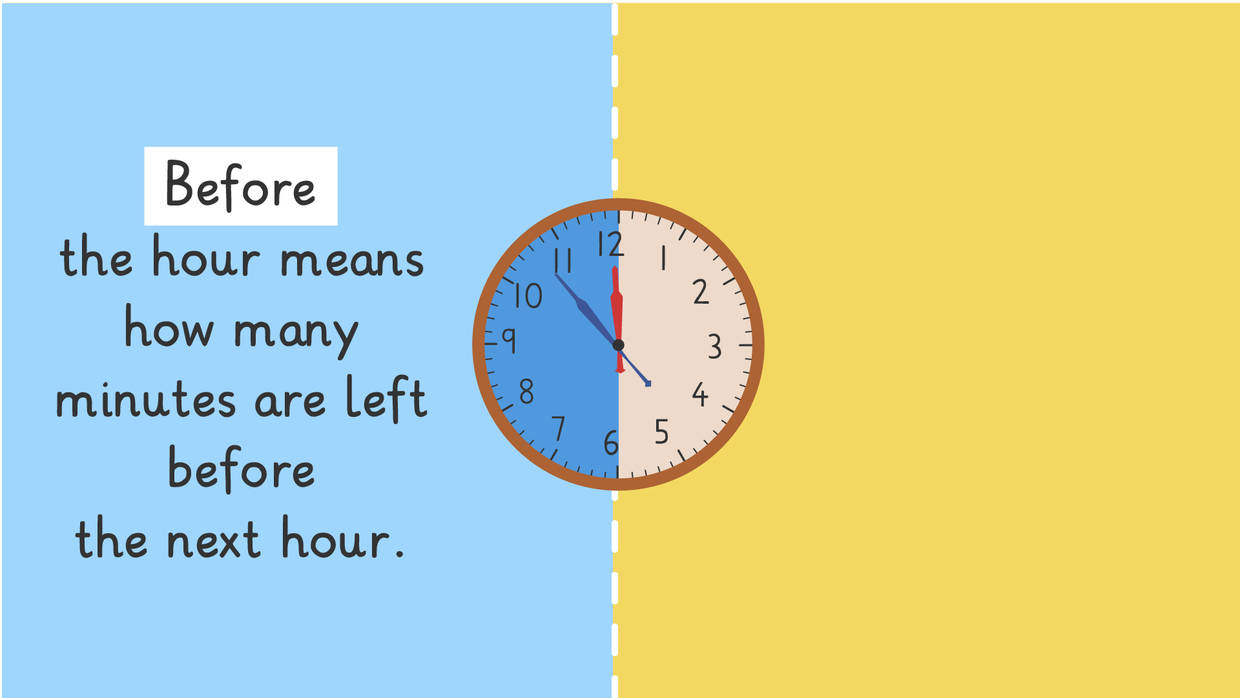#### How can we Tell Time to the Nearest Minute using Before the Hour and After the Hour?- Example

The clock below shows what time the Monster Truck show starts, but how do you tell time to the minute using before the hour or after the hour?Let’s start by asking, is the minute hand on the right side of the clock or the left side? In this example, the minute hand is on the right side so we will use the expression after the hour.

Next, we will count how many minutes have passed in the hour so far. If you count the tick marks on the clock from the beginning of the hour to where the minute hand is, you will count six, so we know it is six minutes after the hour.

Lastly, we can determine the hour by looking at the hour hand. The hour hand is on ten, so the Monster Truck show starts at six minutes after ten.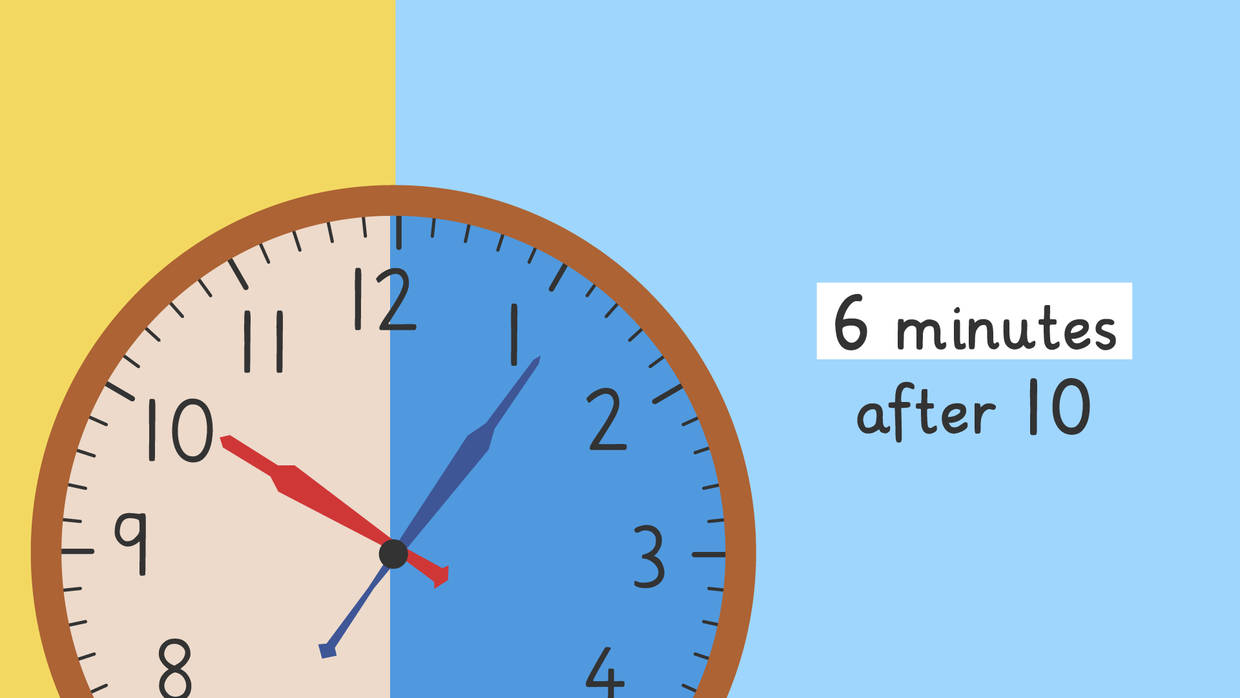Now let’s see what time the Amazing Acrobats show starts. Do we use the expression before the hour or after the hour?Let’s start by asking, is the minute hand on the right side of the clock or the left side? In this example, the minute hand is on the left side so we will use the expression before the hour.

Next, we will count how many minutes are left before the next hour. If you count the tick marks on the clock from where the minute hand is to the end of the hour, you will count seven, so we know it is seven minutes before the next hour.

Lastly, we can determine the hour by looking at the hour hand. The last number the hour hand passed is eleven, so the Amazing Acrobats show starts at seven minutes before twelve.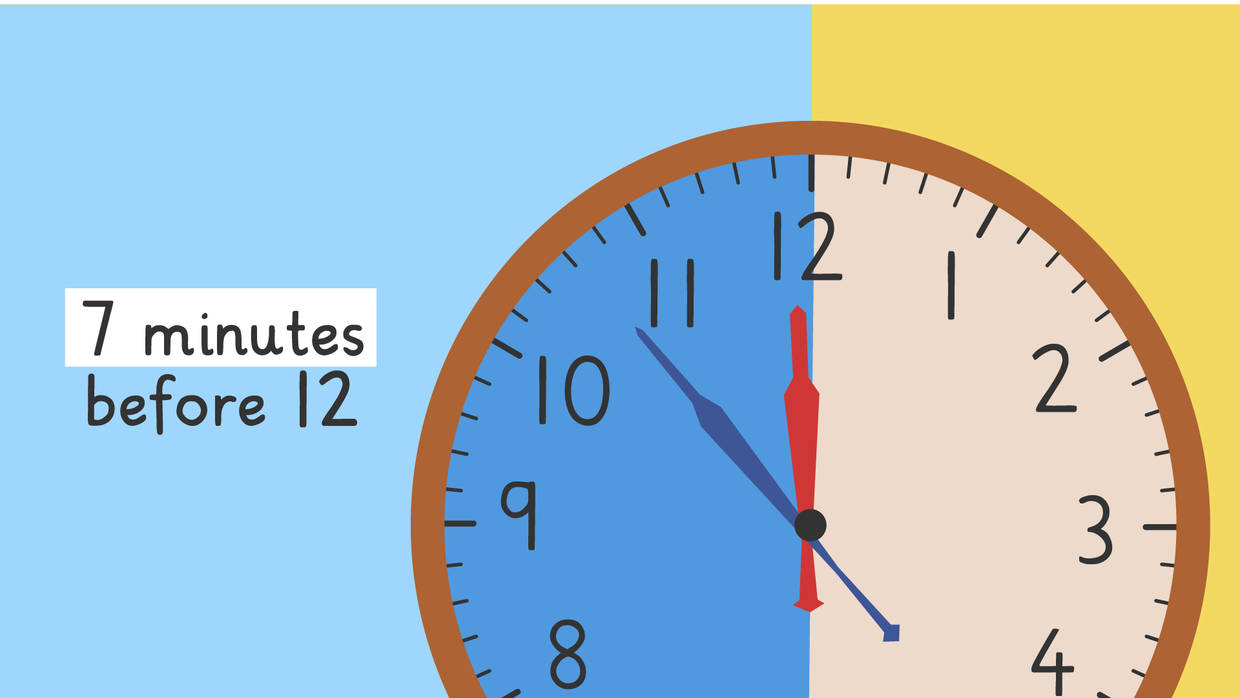#### How can we Tell Time to the Minute using Before the Hour and After the Hour? - Summary

Remember, when we tell time to the minute using the expressions before the hour and after the hour

• Step 1: Identify which expression to use
• Step 2: Count the minutes
• Step 3: Determine the hour

## Telling Time to the Minute: Before and After the Hour Exercise

Would you like to practice what you’ve just learned? Practice problems for this video Telling Time to the Minute: Before and After the Hour help you practice and recap your knowledge.
• ### Sort the steps to tell time before and after the hour.

Hints

Think back! What should you do before you count the minutes before the hour?

Before you determine the hour, what must you do?

Solution
1. Divide clock in half.
2. Which side is the minute hand on?
3. Count the minutes before the hour.
4. Lastly, determine the hour.
• ### Find the clock that matches the time.

Hints

Remember after the hour means that the minute hand is on the right and before the hour means that the minute hand is on the left.

Count the minutes before or after the hour.

Determine the hour by looking if the hour hand is before the next hour or after the next hour.

Solution

• The minute hand is on the left side, so we can use the expression before the hour.
After skip counting and counting up the minutes until the next hour, we get 12 minutes. Since the hour hand is right before 6, we know it is 12 minutes before 6. _____________________________________________________________________________________ For the other clocks:
• The minute hand is on the right side, so we can use the expression after the hour.
After skip counting and counting up the minutes after the hour, we get 22 minutes. Since the hour hand is right after 3, we know it is 22 minutes after 3.
• The minute hand is on the left side, so we can use the expression before the hour.
After skip counting and counting up the minutes until the next hour, we get 7 minutes. Since the hour hand is right before 12, we know it is 7 minutes before 12.
• The minute hand is on the right side, so we can use the expression after the hour.
After skip counting and counting up the minutes after the hour, we get 6 minutes. Since the hour hand is right after 10, we know it is 6 minutes after 10.

• ### Match the time to the clock.

Hints

Remember after the hour means that the minute hand is on the right and before the hour means that the minute hand is on the left.

Count the minutes before or after the hour.

Determine the hour by looking if the hour hand is before the next hour or after the next hour.

Solution

The first clock:

• The minute hand is on the right side, so we can use the expression after the hour.
• After skip counting and counting up the minutes after the hour, we get 18 minutes.
• Since the hour hand is right after 4, we know it is 18 minutes after 4.
_____________________________________________________________________________________ For the other clocks:
The second clock:
• The minute hand is on the left side, so we can use the expression before the hour.
• After skip counting and counting up the minutes until the next hour, we get 28 minutes.
• Since the hour hand is right before 10, we know it is 28 minutes before 10.
The third clock:
• The minute hand is on the right side, so we can use the expression after the hour.
• After skip counting and counting up the minutes after the hour, we get 8 minutes.
• Since the hour hand is right after 6, we know it is 8 minutes after 6.
The last clock:
• The minute hand is on the left side, so we can use the expression before the hour.
• After skip counting and counting up the minutes until the next hour, we get 17 minutes.
• Since the hour hand is right before 8, we know it is 17 minutes before 8.

• ### What time is it before or after the hour?

Hints

Remember after the hour means that the minute hand is on the right and before the hour means that the minute hand is on the left.

Count the minutes before or after the hour.

Determine the hour by looking if the hour hand is before the next hour or after the next hour.

Solution

The first clock:

• The minute hand is on the left side, so we can use the expression before the hour.
• After skip counting and counting up the minutes until the next hour, we get 18 minutes.
• Since the hour hand is right before 2, we know it is 18 minutes before 2.
_____________________________________________________________________________________ For the other clocks:
The second clock:
• The minute hand is on the right side, so we can use the expression after the hour.
• After skip counting and counting up the minutes after the hour, we get 16 minutes.
• Since the hour hand is right after 10, we know it is 16 minutes after 10.
The third clock:
• The minute hand is on the left side, so we can use the expression before the hour.
• After skip counting and counting up the minutes until the next hour, we get 3 minutes.
• Since the hour hand is right before 6, we know it is 3 minutes before 6.
The last clock:
• The minute hand is on the right side, so we can use the expression after the hour.
• After skip counting and counting up the minutes after the hour, we get 8 minutes.
• Since the hour hand is right after 8, we know it is 8 minutes after 8.

• ### Identify which expression to use.

Hints

Remember, the clock is divided in half at the 30 minute mark.

Remember, after the hour means that the minute hand is on the right, and before the hour means that the minute hand is on the left.

Solution

The clock is divided in half at the 30 minute mark. Before the hour is used when the minute hand is on the left. After the hour is used when the minute hand is on the right.

• The first clock is after the hour because the minute hand is on the right.
• The second clock is before the hour because the minute hand is on the left.
• The third clock is after the hour because the minute hand is on the right.
• The last clock is before the hour because the minute hand is on the left.

• ### Which times are wrong?

Hints

Remember after the hour means that the minute hand is on the right and before the hour means that the minute hand is on the left.

Count the minutes before or after the hour.

Determine the hour by looking if the hour hand is before the next hour or after the next hour.

Solution

The second time is 7 minutes before 6, not 7 minutes before 5.

• The minute hand is on the left side, so we can use the expression before the hour.
• After skip counting and counting up the minutes until the next hour, we get 7 minutes.
• Since the hour hand is right before 6, we know it is 7 minutes before 6.
________________________________________________________________________________________________________ For the other clocks:
The first time is 26 minutes after 1, not 26 minutes before 1.
• The minute hand is on the right side, so we can use the expression after the hour.
• After skip counting and counting up the minutes after the hour, we get 26 minutes.
• Since the hour hand is right after 1, we know it is 26 minutes after 1.
The third time is 16 minutes after 8, not 16 minutes before 4.
• The minute hand is on the right side, so we can use the expression after the hour.
• After skip counting and counting up the minutes after the hour, we get 16 minutes.
• Since the hour hand is right after 8, we know it is 16 minutes after 8.
The last time is 3 minutes after 11, not 3 minutes after 12.
• The minute hand is on the right side, so we can use the expression after the hour.
• After skip counting and counting up the minutes after the hour, we get 3 minutes.
• Since the hour hand is right after 11, we know it is 3 minutes after 11.